The GLM Procedure
 OUTPUT Statement
OUTPUT <OUT=SAS-data-set> keyword=names <...keyword=names> <option> ;

The OUTPUT statement creates a new SAS data set that saves diagnostic measures calculated after fitting the model. At least one specification of the form keyword=names is required.

All the variables in the original data set are included in the new data set, along with variables created in the OUTPUT statement. These new variables contain the values of a variety of diagnostic measures that are calculated for each observation in the data set. If you want to create a permanent SAS data set, you must specify a two-level name (see SAS Language Reference: Concepts for more information about permanent SAS data sets).

Details on the specifications in the OUTPUT statement follow.

keyword=names

specifies the statistics to include in the output data set and provides names to the new variables that contain the statistics. Specify a keyword for each desired statistic (see the following list of keywords), an equal sign, and the variable or variables to contain the statistic.

In the output data set, the first variable listed after a keyword in the OUTPUT statement contains that statistic for the first dependent variable listed in the MODEL statement; the second variable contains the statistic for the second dependent variable in the MODEL statement, and so on. The list of variables following the equal sign can be shorter than the list of dependent variables in the MODEL statement. In this case, the procedure creates the new names in order of the dependent variables in the MODEL statement. See the section Examples.

The keywords allowed and the statistics they represent are as follows:

COOKD

Cook’s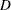influence statistic

COVRATIO

standard influence of observation on covariance of parameter estimates

DFFITS

standard influence of observation on predicted value

H

leverage,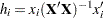LCL

lower bound of a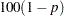% confidence interval for an individual prediction. The-level is equal to the value of the ALPHA= option in the OUTPUT statement or, if this option is not specified, to the ALPHA= option in the PROC GLM statement. If neither of these options is set, then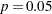by default, resulting in the lower bound for a 95% confidence interval. The interval also depends on the variance of the error, as well as the variance of the parameter estimates. For the corresponding upper bound, see the UCL keyword.

LCLM

lower bound of a% confidence interval for the expected value (mean) of the predicted value. The-level is equal to the value of the ALPHA= option in the OUTPUT statement or, if this option is not specified, to the ALPHA= option in the PROC GLM statement. If neither of these options is set, thenby default, resulting in the lower bound for a 95% confidence interval. For the corresponding upper bound, see the UCLM keyword.

PREDICTED | P

predicted values

PRESS

residual for theth observation that results from dropping it and predicting it on the basis of all other observations. This is the residual divided by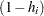, where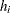is the leverage, defined previously.

RESIDUAL | R

residuals, calculated as ACTUALPREDICTED

RSTUDENT

a studentized residual with the current observation deleted

STDI

standard error of the individual predicted value

STDP

standard error of the mean predicted value

STDR

standard error of the residual

STUDENT

studentized residuals, the residual divided by its standard error

UCL

upper bound of a% confidence interval for an individual prediction. The-level is equal to the value of the ALPHA= option in the OUTPUT statement or, if this option is not specified, to the ALPHA= option in the PROC GLM statement. If neither of these options is set, thenby default, resulting in the upper bound for a 95% confidence interval. The interval also depends on the variance of the error, as well as the variance of the parameter estimates. For the corresponding lower bound, see the LCL keyword.

UCLM

upper bound of a% confidence interval for the expected value (mean) of the predicted value. The-level is equal to the value of the ALPHA= option in the OUTPUT statement or, if this option is not specified, to the ALPHA= option in the PROC GLM statement. If neither of these options is set, thenby default, resulting in the upper bound for a 95% confidence interval. For the corresponding lower bound, see the LCLM keyword.

OUT=SAS-data-set

gives the name of the new data set. By default, the procedure uses the DATAconvention to name the new data set.

The following option is available in the OUTPUT statement and is specified after a slash (/):

ALPHA=p

specifies the level of significancefor% confidence intervals. By default,is equal to the value of the ALPHA= option in the PROC GLM statement or 0.05 if that option is not specified. You can use values between 0 and 1.

See Chapter 4, Introduction to Regression Procedures, and the section Influence Statistics in Chapter 74, The REG Procedure, for details on the calculation of these statistics.

### Examples

The following statements show the syntax for creating an output data set with a single dependent variable.

```proc glm;
class a b;
model y=a b a*b;
output out=new p=yhat r=resid stdr=eresid;
run;
```

These statements create an output data set named new. In addition to all the variables from the original data set, new contains the variable yhat, with values that are predicted values of the dependent variable y; the variable resid, with values that are the residual values of y; and the variable eresid, with values that are the standard errors of the residuals.

The following statements show a situation with five dependent variables.

```proc glm;
by group;
class a;
model y1-y5=a x(a);
output out=pout predicted=py1-py5;
run;
```

The data set pout contains five new variables, py1 through py5. The values of py1 are the predicted values of y1; the values of py2 are the predicted values of y2; and so on.

For more information about the data set produced by the OUTPUT statement, see the section Output Data Sets.Previous Page | Next Page | Top of Page